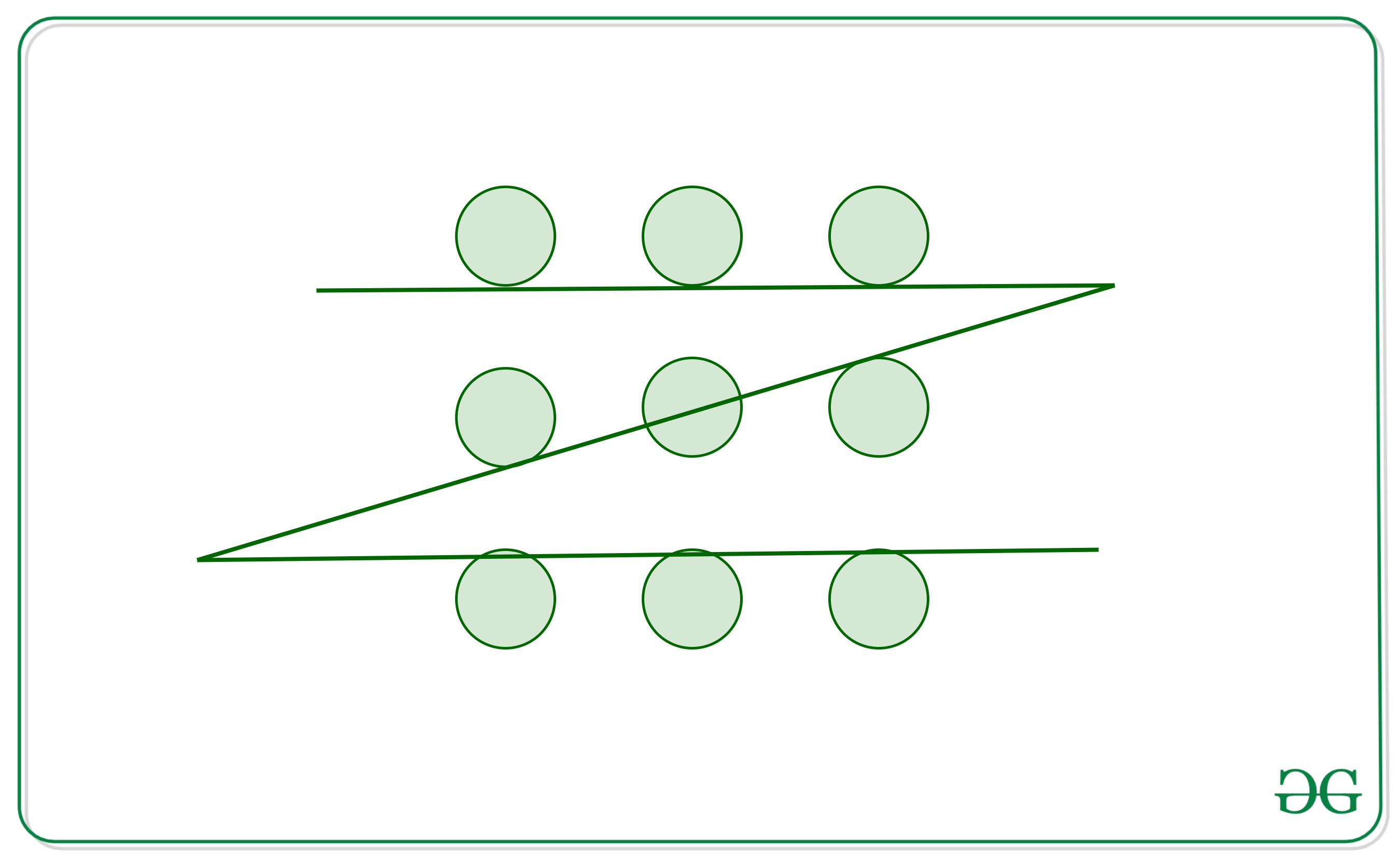Open in App
Not now

# Puzzle | Connect 9 circles each arranged at center of a Matrix using 3 straight lines

• Difficulty Level : Medium
• Last Updated : 18 Jan, 2023

Consider 9 circles each arranged at the center of cells of a 2-D matrix of shape 3*3. Draw 3 straight lines without removing pen from paper such that each of the 9 circles is in contact with at least one line.

Solution:
A similar problem is present here Connect 9 dots using 4 lines.
The difference from above problem is that here there are 9 circles not 9 points. Also we can only draw 3 lines.
One such way of doing what is asked is:The thing to notice is that since these are circles, not points, we can draw a line that is tangent to the circle. There is no need to pass the line through the center as the only contact with the circle is required.

My Personal Notes arrow_drop_up